#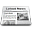Deep Learning of MNIST handwritten digits

In this document I am going create a video showing the training of the inner-most layer of Deep Belief Network (DBN) using the MNIST dataset of handwritten digits. I will use our `DeepLearning` R package that implements flexible DBN architectures with an object-oriented interface.

## MNIST

The MNIST dataset is a database of handwritten digits with 60,000 training images and 10,000 testing images. You can learn everything about it on Wikipedia. In short, it is the go-to dataset to train and test handwritten digit recognition machine learning algorithms.

I made an R package for easy access, named `mnist`. The easiest way to install it is with `devtools`. If you don't have it already, let's first install `devtools`:

```if (!require("devtools")) {install.packages("devtools")}
```

Now we can install `mnist`:

```devtools::install_github("xrobin/mnist")
```

## PCA

In order to see what the dataset looks like, let's use PCA to reduce it to two dimensions.

```pca <- prcomp(mnist\$train\$x)
plot.mnist(
prediction = predict(pca, mnist\$test\$x),
reconstruction = tcrossprod(
predict(pca, mnist\$test\$x)[,1:2], pca\$rotation[,1:2]),
highlight.digits = c(72, 3, 83, 91, 6688, 7860, 92, 1, 180, 13))
```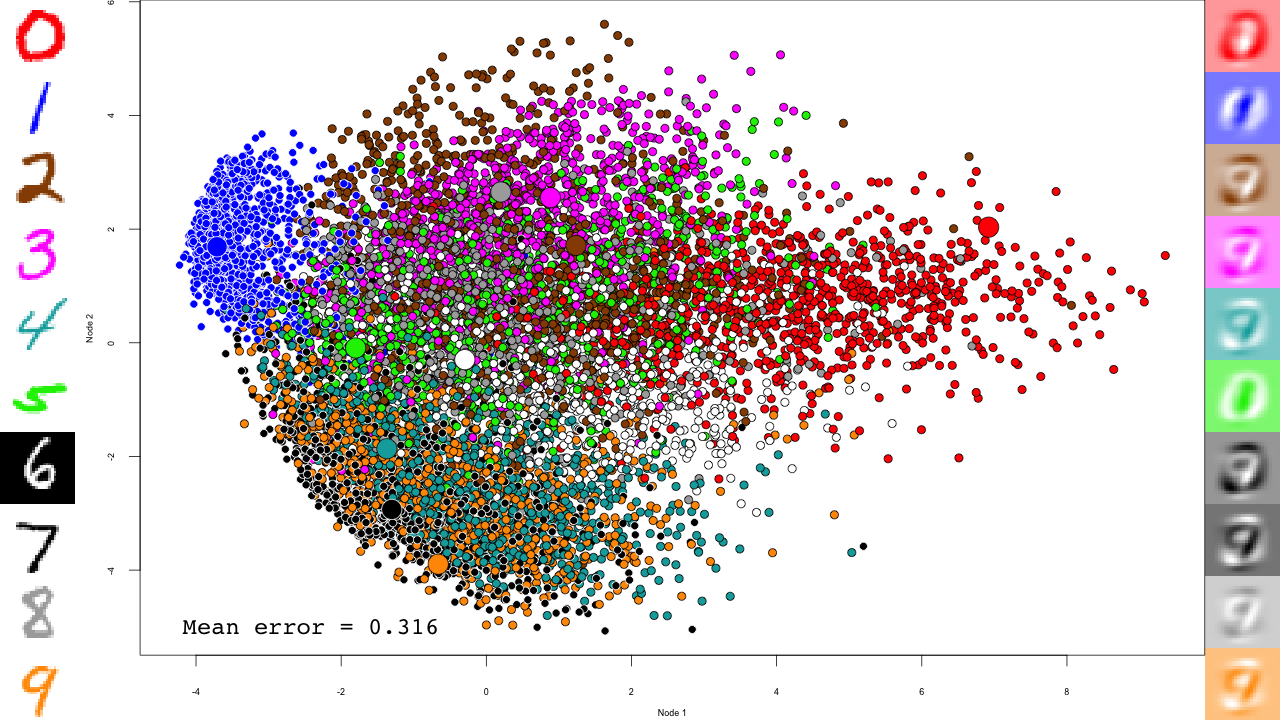Let's take a minute to describe this plot. The central scatterplot shows first two components of the PCA of all digits in the test set. On the left hand side, I picked 10 representative digits from the test set to highlight, which are shown as larger circles in the central scatterplot. On the left are the "reconstructed digits", which were reconstructed from the two first dimensions of the PCA. While we can see some digit-like structures, it is basically impossible to recognize them. We can see some separation of the digits in the 2D space as well, but it is pretty weak and some pairs cannot be distinguished at all (like 4 and 9). Of course the reconstructions would look much better had we kept all PCA dimensions, but so much for dimensionality reduction.

## Deep Learning

Now let's see if we can do better with Deep Learning. We'll use a classical Deep Belief Network (DBN), based on Restricted Boltzmann Machines (RBM) similar to what Hinton described back in 2006 (Hinton & Salakhutdinov, 2006). The training happens in two steps: a pre-training step with contrastive divergence stochastic gradient descent brings the network to a reasonable starting point for a more conventional conjugate gradient optimization (hereafter referred to as fine-tuning).

I implemented this algorithm with a few modifications in an R package which is available on GitHub. The core of the processing is done in C++ with RcppEigen (Bates & Eddelbuettel, 2013) for higher speed. Using `devtools` again:

```devtools::install_github("xrobin/DeepLearning")
```

We will use this code to train a 5 layers deep network, that reduces the digits to an abstract, 2D representation. By looking at this last layer throughout the training process we can start to understand how the network learns to recognize digits. Let's start by loading the required packages and the MNIST dataset, and create the DBN.

```library(DeepLearning)
library(mnist)
data(mnist)

dbn <- DeepBeliefNet(Layers(c(784, 1000, 500, 250, 2),
input = "continuous", output = "gaussian"),
initialize = "0")
```

We just created the 5-layers DBN, with continuous, 784 nodes input (the digit image pixels), and a 2 nodes, gaussian output. It is initialized with 0, but we could have left out the `initialize` to start from a random initilization (Bengio et al., 2007). Before we go, let's define a few useful variables:

```output.folder <- "video" # Where to save the output
maxiters.pretrain <- 1e6  # Number of pre-training iterations
maxiters.train <- 10000 # Number of fine-tuning iterations
run.training <- run.images <- TRUE # Turn any of these off
# Which digits to highlight and reconstruct
highlight.digits = c(72, 3, 83, 91, 6688, 7860, 92, 1, 180, 13)
```

We'll also need the following function to show the elapsed time:

```format.timediff <- function(start.time) {
diff = as.numeric(difftime(Sys.time(), start.time, units="mins"))
hr <- diff%/%60
min <- floor(diff - hr * 60)
sec <- round(diff%%1 * 60,digits=2)
return(paste(hr,min,sec,sep=':'))
}
```

## Pre-training

Initially, the network is a stack of RBMs that we need to pre-train one by one. Hinton & Salakhutdinov (2006) showed that this step is critical to train deep networks. We will use 1000000 iterations (`maxiters.pretrain`) of contrastive divergence, which takes a couple of days on a modern CPU. Let's start with the first three RBMs:

### First three RBMs

```if (run.training) {
sprintf.fmt.iter <- sprintf("%%0%dd", nchar(sprintf("%d", maxiters.pretrain)))

mnist.data.layer <- mnist
for (i in 1:3) {
```

We define a `diag` function that will simply print where we are in the training. Because this function will be called a million times (`maxiters.pretrain`), we can use `rate = "accelerate"` to slow down the printing over time and save a few CPU cycles.

```		diag <- list(rate = "accelerate", data = NULL, f = function(rbm, batch, data, iter, batchsize, maxiters, layer) {
print(sprintf("%s[%s/%s] in %s", layer, iter, maxiters, format.timediff(start.time)))
})
```

We can get the current RBM, and we will work on it directly. Let's save it for good measure, as well as the current time for the progress function:

```		rbm <- dbn[[i]]
save(rbm, file = file.path(output.folder, sprintf("rbm-%s-%s.RData", i, "initial")))
start.time <- Sys.time()
```

Now we can start the actual pre-training:

```		rbm <- pretrain(rbm, mnist.data.layer\$train\$x,
penalization = "l2", lambda=0.0002, momentum = c(0.5, 0.9),
epsilon=c(.1, .1, .1, .001)[i], batchsize = 100, maxiters=maxiters.pretrain,
continue.function = continue.function.always, diag = diag)
```

This can take some time, especially for the first layers which are larger. Once it is done, we predict the data through this RBM for the next layer and save the results:

```		mnist.data.layer\$train\$x <- predict(rbm, mnist.data.layer\$train\$x)
mnist.data.layer\$test\$x <- predict(rbm, mnist.data.layer\$test\$x)
save(rbm, file = file.path(output.folder, sprintf("rbm-%s-%s.RData", i, "final")))
dbn[[i]] <- rbm
}
```

### Last RBM

This is very similar to the previous three, but note that we save the RBM within the `diag` function. We could generate the plot directly, but it is easier to do it later once we have some idea about the final axis we will need. Please note the `rate = "accelerate"` here. You probably don't want to save a million RBM objects on your hard drive, both for speed and space reasons.

```	rbm <- dbn[]
diag <- list(rate = "accelerate", data = NULL, f = function(rbm, batch, data, iter, batchsize, maxiters, layer) {
save(rbm, file = file.path(output.folder, sprintf("rbm-4-%s.RData", sprintf(sprintf.fmt.iter, iter))))
print(sprintf("%s[%s/%s] in %s", layer, iter, maxiters, format.timediff(start.time)))
})
save(rbm, file = file.path(output.folder, sprintf("rbm-%s-%s.RData", 4, "initial")))
start.time <- Sys.time()
rbm <- pretrain(rbm, mnist.data.layer\$train\$x,  penalization = "l2", lambda=0.0002,
epsilon=.001, batchsize = 100, maxiters=maxiters.pretrain,
continue.function = continue.function.always, diag = diag)
save(rbm, file = file.path(output.folder, sprintf("rbm-4-%s.RData", "final")))
dbn[] <- rbm
```

If we were not querying the last layer, we could have pre-trained the entire network at once with the following call:

```	dbn <- pretrain(dbn, mnist.data.layer\$train\$x,
penalization = "l2", lambda=0.0002, momentum = c(0.5, 0.9),
epsilon=c(.1, .1, .1, .001), batchsize = 100,
maxiters=maxiters.pretrain,
continue.function = continue.function.always)
```

### Pre-training parameters

Pre-training RBMs is quite sensitive to the use of proper parameters. With improper parameters, the network can quickly go crazy and start to generate infinite values. If that happens to you, you should try to tune one of the following parameters:

• `penalization`: this is the penalty of introducing or increasing the value of a weight. We used L2 regularization, but `"l1"` is available if a sparser weight matrix is needed.
• `lambda`: the regularization rate. In our experience 0.0002 works fine with the MNIST and other datasets of similar sizes such as cellular imaging data. Too small or large values will result in over- or under-fitted networks, respectively.
• `momentum`: helps avoiding oscillatory behaviors, where the network oscillate between iterations. Allowed values can range from 0 (no momentum) to 1 (full momentum = no training). Here we used an increasing gradient of momentum which starts at 0.5 and increases linearly to 0.9, in order to stabilize the final network without compromising early training steps.
• `epsilon`: the learning rate. Typically, 0.1 works well with binary and continuous output layers, and must be decreased to around 0.001 for gaussian outputs. Too large values will drive the network to generate infinities, while too small ones will slow down the training.
• `batchsize`: larger batch sizes will result in smoother but slower training. Small batch sizes will make the training "jumpy", which can be compensated by lower learning rates (epsilon) or increased momentum.

## Fine-tuning

This is where the real training happens. We use conjugate gradients to find the optimal solution. Again, the `diag` function saves the DBN. This time we use `rate = "each"` to save every step of the training. First we have way fewer steps, but also the training itself happen at a much more stable speed than in the pre-training, where things slow down dramatically.

```	sprintf.fmt.iter <- sprintf("%%0%dd", nchar(sprintf("%d", maxiters.train)))
diag <- list(rate = "each", data = NULL, f = function(dbn, batch, data, iter, batchsize, maxiters) {
save(dbn, file = file.path(output.folder, sprintf("dbn-finetune-%s.RData", sprintf(sprintf.fmt.iter, iter))))
print(sprintf("[%s/%s] in %s", iter, maxiters, format.timediff(start.time)))
})
save(dbn, file = file.path(output.folder, sprintf("dbn-finetune-%s.RData", "initial")))
start.time <- Sys.time()
dbn <- train(unroll(dbn), mnist\$train\$x, batchsize = 100, maxiters=maxiters.train,
continue.function = continue.function.always, diag = diag)
save(dbn, file = file.path(output.folder, sprintf("dbn-finetune-%s.RData", "final")))
}
```

And that's it, our DBN is now fully trained!

## Generating the images

Now we need to read in the saved network states again, pass the data through the network (`predict`) and save this in HD-sized PNG file.

The first three RBMs are only loaded into the DBN

```if (run.images) {
for (i in 1:3) {
dbn[[i]] <- rbm
}
```

The last RBM is where interesting things happen.

```	for (file in list.files(output.folder, pattern = "rbm-4-.+\\.RData", full.names = TRUE)) {
print(file)
dbn[] <- rbm
iter <- stringr::str_match(file, "rbm-4-(.+)\\.RData")[,2]
```

We now predict and reconstruct the data, and calculate the mean reconstruction error:

```		predictions <- predict(dbn, mnist\$test\$x)
reconstructions <- reconstruct(dbn, mnist\$test\$x)
iteration.error <- errorSum(dbn, mnist\$test\$x) / nrow(mnist\$test\$x)
```

Now the actual plotting. Here I selected `xlim` and `ylim` values that worked well for my training run, but your mileage may vary.

```		png(sub(".RData", ".png", file), width = 1280, height = 720) # hd output
plot.mnist(model = dbn, x = mnist\$test\$x, label = mnist\$test\$y+1, predictions = predictions, reconstructions = reconstructions,
ncol = 16, highlight.digits = highlight.digits,
xlim = c(-12.625948, 8.329168), ylim = c(-10.50657, 13.12654))
par(family="mono")
legend("bottomleft", legend = sprintf("Mean error = %.3f", iteration.error), bty="n", cex=3)
legend("bottomright", legend = sprintf("Iteration = %s", iter), bty="n", cex=3)
dev.off()
}
```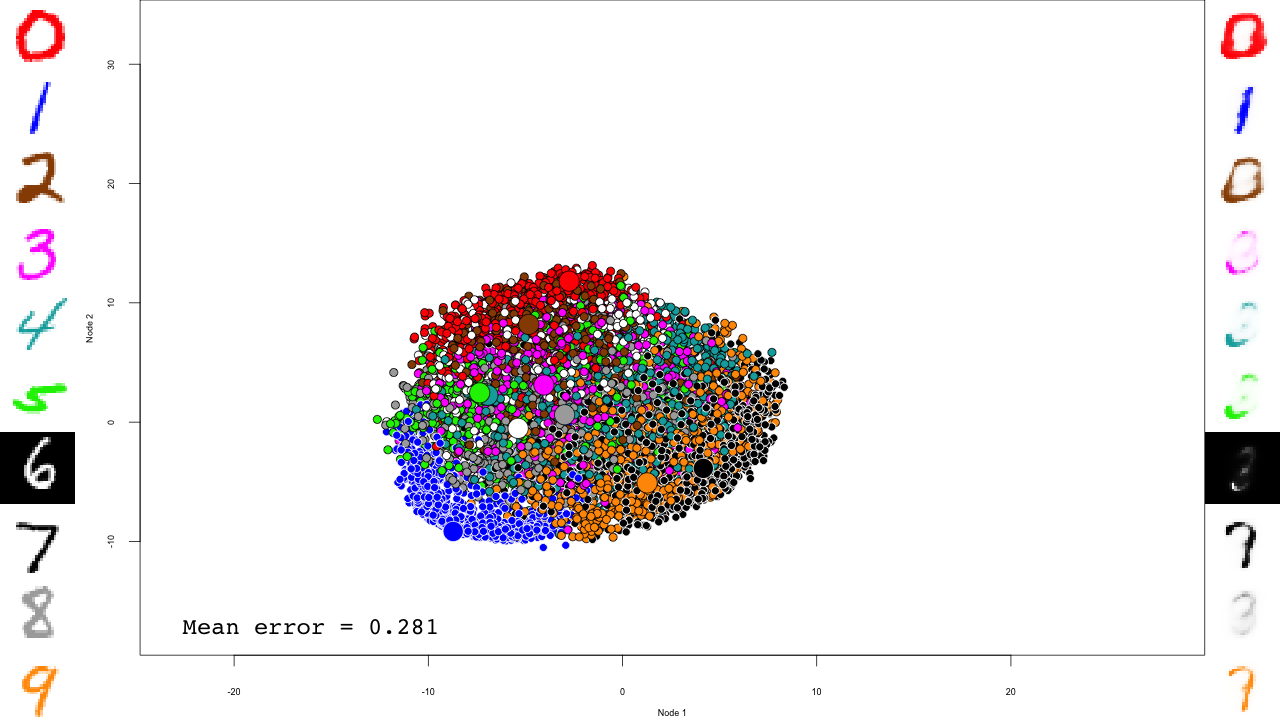We do the same with the fine-tuning:

```	for (file in list.files(output.folder, pattern = "dbn-finetune-.+\\.RData", full.names = TRUE)) {
print(file)
iter <- stringr::str_match(file, "dbn-finetune-(.+)\\.RData")[,2]
predictions <- predict(dbn, mnist\$test\$x)
reconstructions <- reconstruct(dbn, mnist\$test\$x)
iteration.error <- errorSum(dbn, mnist\$test\$x) / nrow(mnist\$test\$x)
png(sub(".RData", ".png", file), width = 1280, height = 720) # hd output
plot.mnist(model = dbn, x = mnist\$test\$x, label = mnist\$test\$y+1, predictions = predictions, reconstructions = reconstructions,
ncol = 16, highlight.digits = highlight.digits,
xlim = c(-22.81098,  27.94829), ylim = c(-17.49874,  33.34688))
par(family="mono")
legend("bottomleft", legend = sprintf("Mean error = %.3f", iteration.error), bty="n", cex=3)
legend("bottomright", legend = sprintf("Iteration = %s", iter), bty="n", cex=3)
dev.off()
}
}
```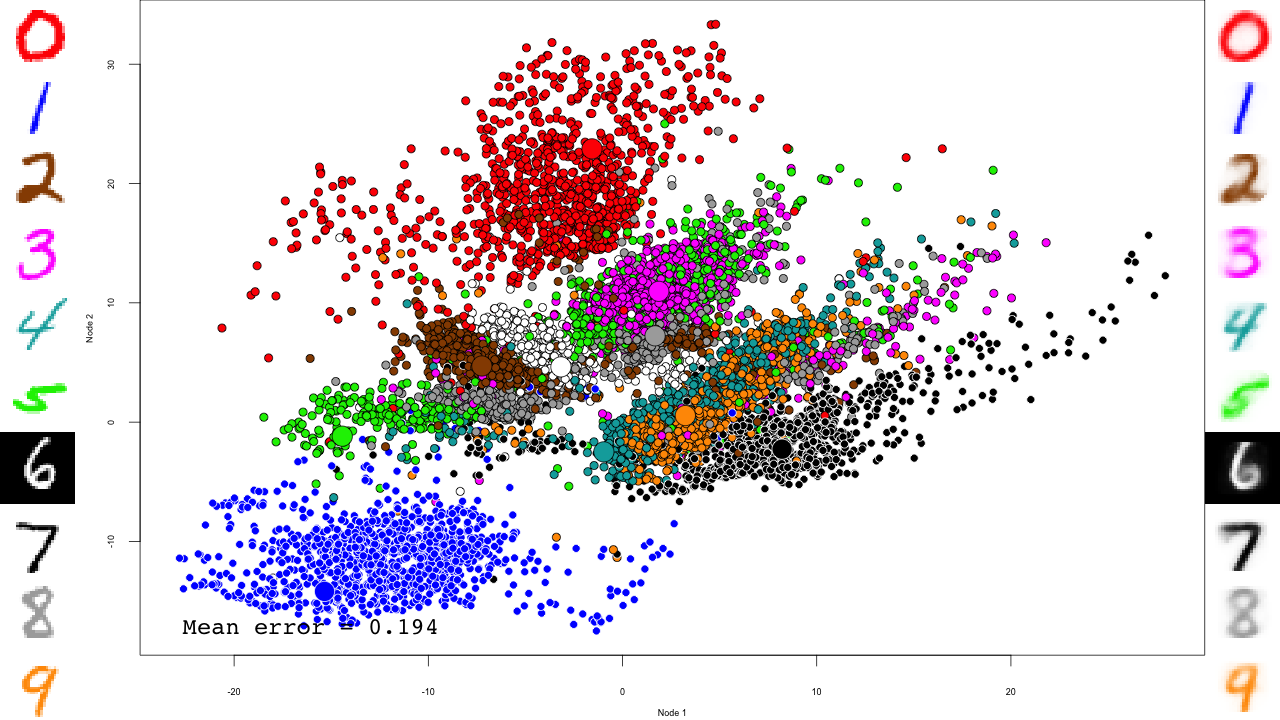## The video

I simply used ffmpeg to convert the PNG files to a video:

```cd video
ffmpeg -pattern_type glob -i "rbm-4-*.png" -b:v 10000000 -y ../rbm-4.mp4
ffmpeg -pattern_type glob -i "dbn-finetune-*.png" -b:v 10000000 -y ../dbn-finetune.mp4
```

And that's it! Notice how the pre-training only brings the network to a state similar to that of a PCA, and the fine-tuning actually does the separation, and how it really makes the reconstructions accurate.

## Application

We used this code to analyze changes in cell morphology upon drug resistance in cancer. With a 27-dimension space, we could describe all of the observed cell morphologies and predict whether a cell was resistant to ErbB-family drugs with an accuracy of 74%. The paper is available in Open Access in Cell Reports, DOI 10.1016/j.celrep.2020.108657.

## Concluding remarks

In this document I described how to build and train a DBN with the `DeepLearning` package. I also showed how to query the internal layer, and use the generative properties to follow the training of the network on handwritten digits.

DBNs have the advantage over Convolutional Networks (CN) that they are fully generative, at least during the pre-training. They are therefore easier to query and interpret as we have demonstrated here. However keep in mind that CNs have demonstrated higher accuracies on computer vision tasks, such as the MNIST dataset.

Additional algorithmic details are available in the `doc` folder of the DeepLearning package.

## References

Our paper, 2021
Longden J., Robin X., Engel M., et al. Deep neural networks identify signaling mechanisms of ErbB-family drug resistance from a continuous cell morphology space. Cell Reports, 2021;34(3):108657.
Bates & Eddelbuettel, 2013
Bates D, Eddelbuettel D. Fast and Elegant Numerical Linear Algebra Using the RcppEigen Package. Journal of Statistical Software, 2013;52(5):1–24.
Bengio et al., 2007
Bengio Y, Lamblin P, Popovici D, Larochelle H. Greedy layer-wise training of deep networks. Advances in neural information processing systems. 2007;19:153–60.
Hinton & Salakhutdinov, 2006
Hinton GE, Salakhutdinov RR. Reducing the Dimensionality of Data with Neural Networks. Science. 2006;313(5786):504–7.

Xavier Robin
Publié le samedi 11 juin 2022 à 16:39 CEST
Lien permanent : /blog/2022/06/11/deep-learning-of-mnist-handwritten-digits
Tags : Programmation
Commentaires : 0

## Commentaires

Aucun commentaire

### Nouveau commentaire

* L'astérisque dénote un champ obligatoire.

En soumettant votre message, vous acceptez qu' il soit publié sous licence CC BY-SA 3.0.

Quelques balises HTML sont autorisées : a[href, hreflang, title], br, em, i, strong, b, tt, samp, kbd, var, abbr[title], acronym[title], code, q[cite], sub, sup.

##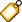Tags

Bruit de fond Hobbys Humour Informatique Internet Livres Logiciels Moi Mon site web Mozilla Photo Politique Programmation Scolaire Ubuntu pROC

##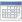Calendrier

lun.mar.mer.jeu.ven.sam.dim.
12345
6789101112
13141516171819
20212223242526
27282930